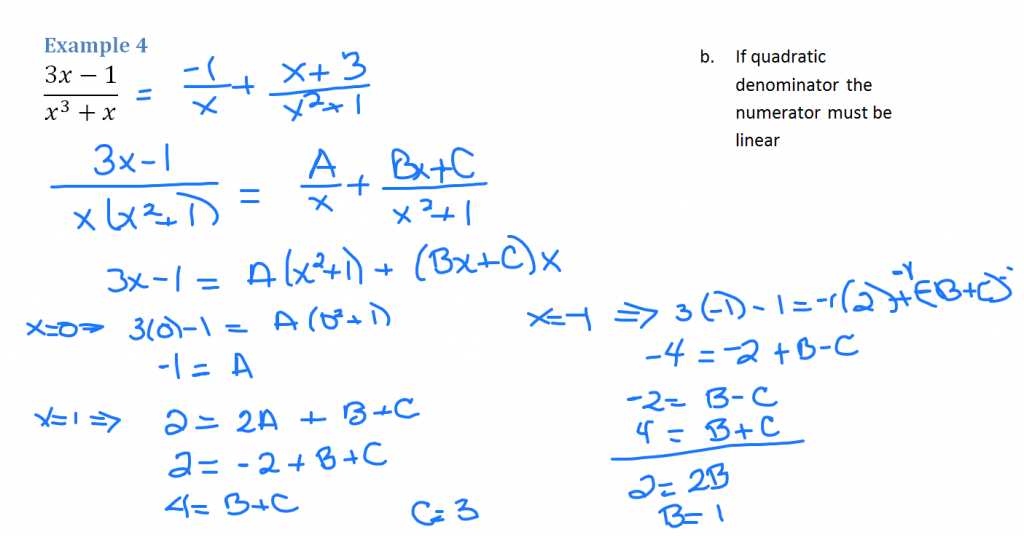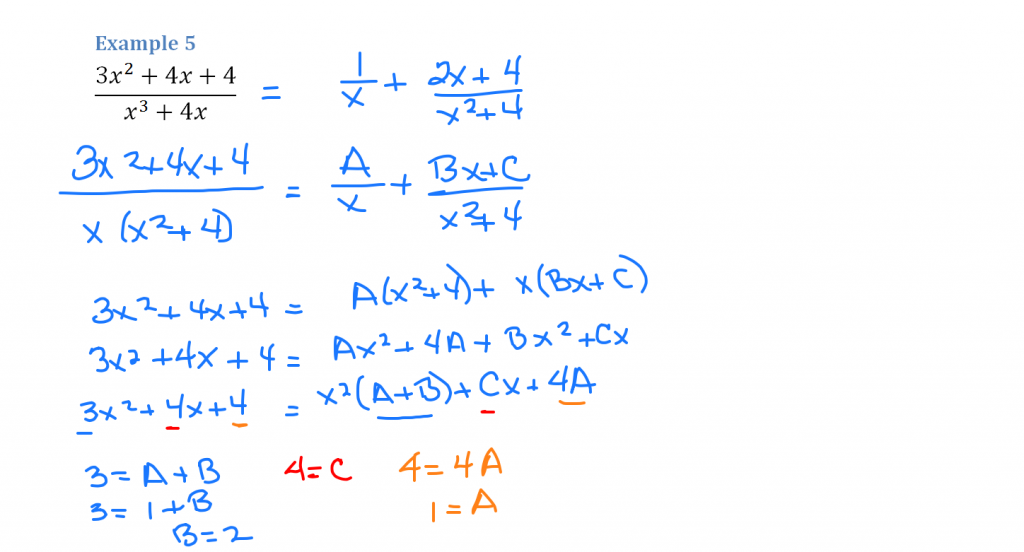# Partial Fractions

//Partial Fractions
Partial Fractions 2017-11-13T22:01:52+00:00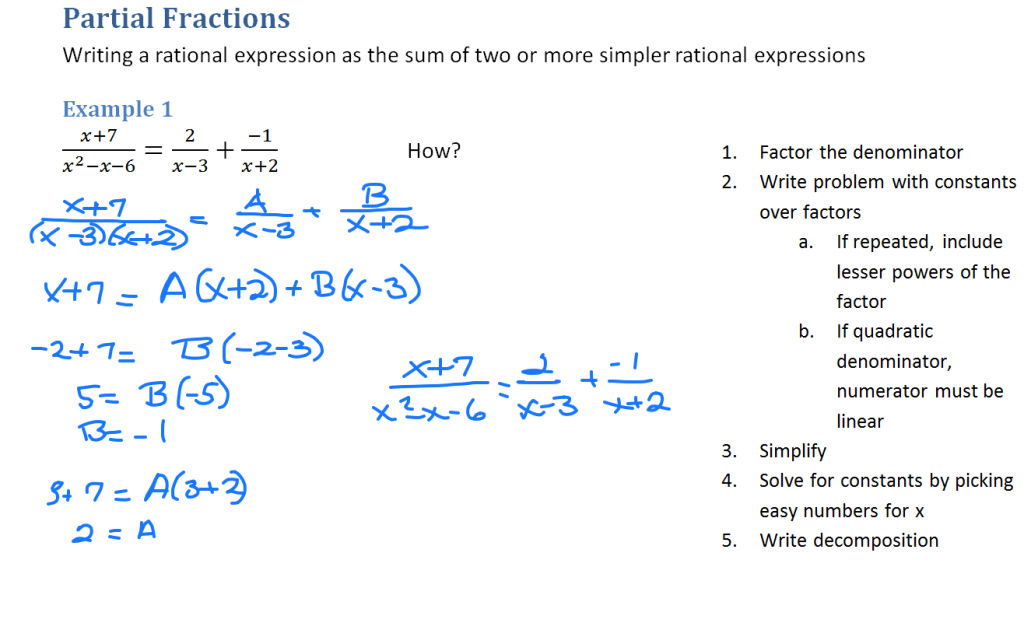Start by rewriting the fraction with its factored denominator. Set it equal to some number A over its first factor plus a number B over its second factor. Simplify by multiplying each term by the LCM. The left side becomes x + 7. On the right you will have A(x + 2) plus B(x – 3).

To simplify, substitute numbers for x that will make zeros in the equation. If x is -2, A disappears and you are left with 5 = B(-5) so B = -1. To eliminate B from the equation, let x be 3. Subbing in 3 for x leaves 10 = 5A so A = 2. Now re-write the partial fraction.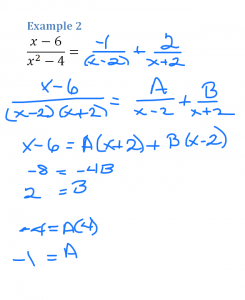Factor the denominator. Write as partial fractions with constants A and B. Multiply each term by the LCM. Then pick convenient values for x that eliminate each constant in turn. When x = -2, the A is eliminated. When x = 2, the B is eliminated. Re-write with the constants in place.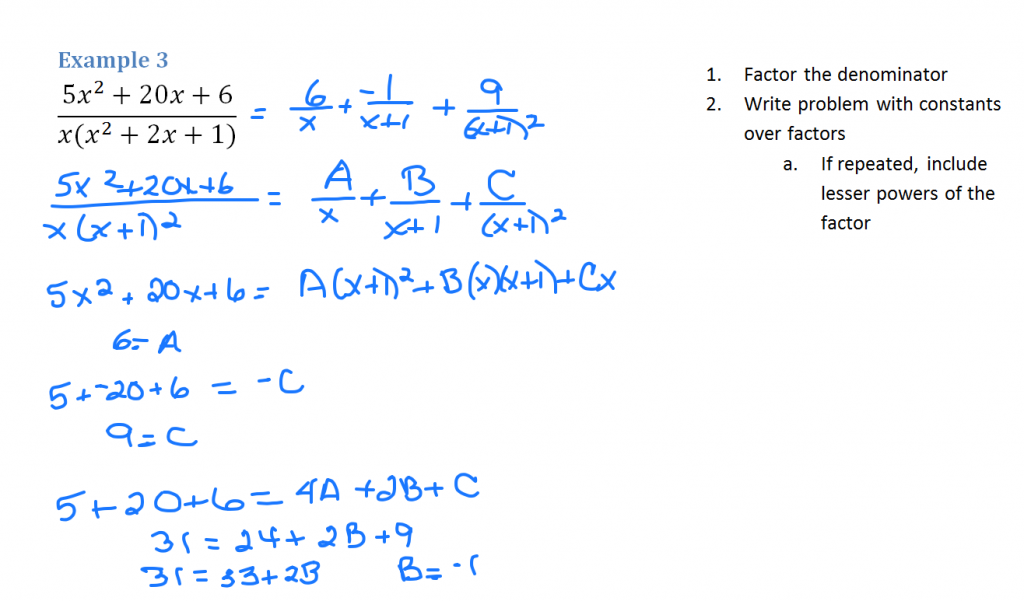Follow the same process, but because the denominator factors into (x+1) squared, it has to be repeated with both the squared factor and its lesser factor. We introduce a C constant to our third fraction.

Eliminate the easy ones by substituting 0 for x and also -1 for x. To determine the value of B, substitute 1 for x (along with the values for A and C).